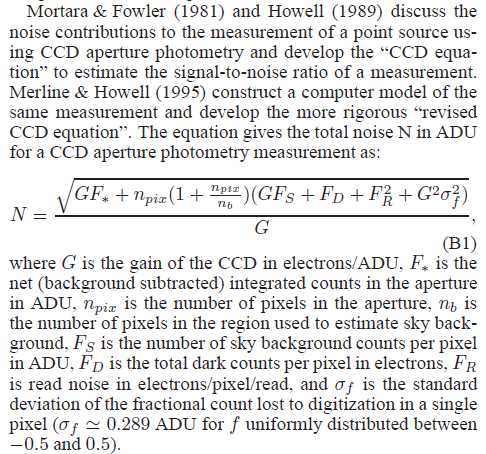# Correct relationship between Read Noise and RMS error?ClassicListThreaded3 messagesOpen this post in threaded view
|

## Correct relationship between Read Noise and RMS error?

 Could you please clear something up for me.  I am trying to determine the relationship between the camera read noise and the RMS error of a typical measurement on a scatter plot (assuming all other factors are equal).  I am not sure if the relationship is linear or square root (or perhaps something else). As an example, assume camera1 has a RN of 9e- and Camera2 has a RN of 1.6e- Using a square root relationship: Camera 1 RMS Error = (RN)^1/2 = (9)^1/2 = 3.0 Camera 2 RMS Error = (RN)^1/2 = (1.6)^1/2 = 1.265 Percent Reduction in RMS Error = (3.0 - 1.265)/3.0 x 100% = 57.84% Assuming a linear relationship: RMS Error Ratio = 1.6/9.0 = 0.178 Percent Reduction in RMS Error = (1 - RMS Error Ratio x 100%) = (1-0.178) x 100% = 82.22% Since we are dealing with a Gaussian distribution, I would assume that the square root model would be more accurate.  Could you please give me your thoughts on this? Sycamore Canyon Observatory https://scosci.com/
 Administrator The noise equation is given by equation B1 in the AIJ paper. Read noise is a squared term that is under a square root, but many other factors are also under the square root.